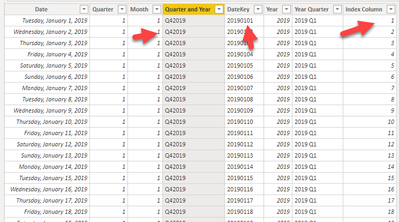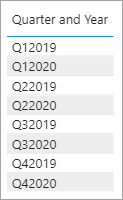cancel
Showing results for
Did you mean:Helper III

## Sort by Quarter not Working with an Index

I am trying to sort data in a stacked graph using a Date Table. I have a column with Dates called Date and a number of data attribute so I can use them in the visuals.

I am trying to get an aggregate set of values by the quarter and their year. What I'm getting is the following error:My Data is arranged like this:How can I get this to sort correctly on a visual? Notice the following:1 ACCEPTED SOLUTIONSuper User

This is the code that creates the table in your model.

``Index Date = CALENDAR (DATE(2019,1,1), DATE(2020,06,30))``

Then you added the calculated columns.  I just changed the code to include the additional fields in the original DAX that creates the table.

So you can

1. Delete all the columns except the date column from your table.
2. Replace the measure that creates the table with mine.

This

``Index Date = CALENDAR (DATE(2019,1,1), DATE(2020,06,30))``

becomes this

``````Index Date =
CALENDAR ( DATE ( 2019, 1, 1 ), DATE ( 2020, 06, 30 ) ),
"Quarter", QUARTER ( [Date] ),
"Month", MONTH ( [Date] ),
"Quarter and Year", "Q" & QUARTER ( [Date] ) & YEAR ( [Date] ),
"DateKey", FORMAT ( [Date], "yyyymmdd" ),
"Year", YEAR ( [Date] ),
"Year Quarter", YEAR ( [Date] ) & " Q" & QUARTER ( [Date] ),
"QYSort", YEAR ( [Date] ) * 100 + QUARTER ( [Date] )
)``````
10 REPLIES 10Super User

``QYSort = YEAR ( [Date] ) * 100 + QUARTER ( [Date] )``

Then you can set the 'Sort By' column on 'Quarter and Year' to be the new 'QSort'

The error is because, for every instance of Q42019 it need 1 and ONLY 1 numeric value in the column you are trying to use as the sort.  Your index column would have 90 different numbers under Q42019.  The QYSort column will give you the single numeric value you need.Helper III

Thank you for your help @jdbuchanan71 . That still didn't work.

I'm learning power BI.

1) I get a circular reference for sorting so it appears there is some persistence on the column sort. I can't seem to delete the sorting except doing a sort ascending on the column?

2) I have these indexes already. See below.  The date column is sorted Ascending. the 'Quarter and Year' field should have a reference already to it.Super User

Here is a sample of the default sort in [Quarter and Year]On the date table I have to:

1. Select the column I want to sort.
2. Click the 'Sort by column' drop down
3. Pick the filed I want to sort byThen the model will sort my filed in the correct order.Helper III

Thank you @jdbuchanan71 . So this appears to be something on the sorting being persisted on the table itself. When you do it appears to work. For me this is the error I get:Super User

No, that means that for some reason it is seeing something like this.

 Quarter and Year QYSort Q1 2019 201901 Q1 2019 201902

Can you save a copy of your .pbix with just the date table and share it here?  Load it to onedrive or drop box and post the link here.Helper III

Here is the .pbisSuper User

Part of the problem is in your [Quarter and Year] column.  If you look at the screen shot the dates cross 2 quarters but they are all tagged Q12019:  The problems is you are taking the QUARTER ( ) of the [Quarter] column.But even with that fixed I was getting circular errors when I should not have been.  Try building the table all in one shot rather than adding the columns one at a time.

``````Index Date =
CALENDAR ( DATE ( 2019, 1, 1 ), DATE ( 2020, 06, 30 ) ),
"Quarter", QUARTER ( [Date] ),
"Month", MONTH ( [Date] ),
"Quarter and Year", "Q" & QUARTER ( [Date] ) & YEAR ( [Date] ),
"DateKey", FORMAT ( [Date], "yyyymmdd" ),
"Year", YEAR ( [Date] ),
"Year Quarter", YEAR ( [Date] ) & " Q" & QUARTER ( [Date] ),
"QYSort", YEAR ( [Date] ) * 100 + QUARTER ( [Date] )
)``````

Then you can add the [Index Column] as a new calculated column although I don't think you need that one.  I was able to get the sorting to work fine in a new book.

I was able to get it to work by deleting all the additional columns you had added then replacing your first measure with the code above.  Then the sorting worked fine.Helper III

Interesting. Where is the code to create the table? Could I have edited that? Is this in query editor?Helper III

@jdbuchanan71 This is the solution. Thanks for this. Now I know to debug the table itself because I think PBI gets confused.Super User

This is the code that creates the table in your model.

``Index Date = CALENDAR (DATE(2019,1,1), DATE(2020,06,30))``

Then you added the calculated columns.  I just changed the code to include the additional fields in the original DAX that creates the table.

So you can

1. Delete all the columns except the date column from your table.
2. Replace the measure that creates the table with mine.

This

``Index Date = CALENDAR (DATE(2019,1,1), DATE(2020,06,30))``

becomes this

``````Index Date =
CALENDAR ( DATE ( 2019, 1, 1 ), DATE ( 2020, 06, 30 ) ),
"Quarter", QUARTER ( [Date] ),
"Month", MONTH ( [Date] ),
"Quarter and Year", "Q" & QUARTER ( [Date] ) & YEAR ( [Date] ),
"DateKey", FORMAT ( [Date], "yyyymmdd" ),
"Year", YEAR ( [Date] ),
"Year Quarter", YEAR ( [Date] ) & " Q" & QUARTER ( [Date] ),
"QYSort", YEAR ( [Date] ) * 100 + QUARTER ( [Date] )
)``````